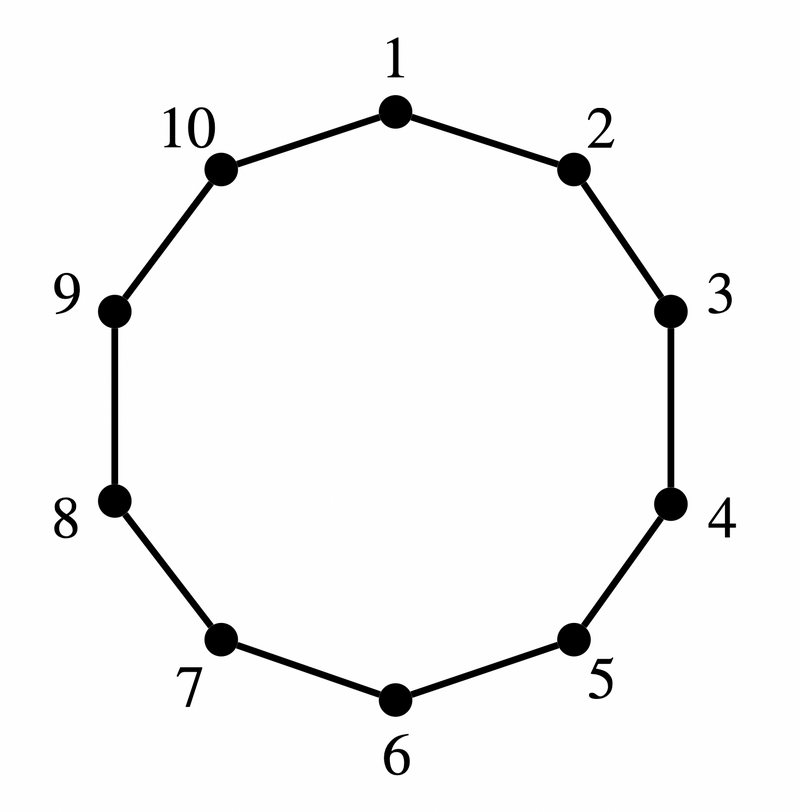﻿ ABC240 A - Edge Checker - Atcoder

# Home

Score : $100$ points

### Problem Statement

In the figure shown in the image below, are the points numbered $a$ and $b$ directly connected by a line segment?### Constraints

• $1 \leq a \lt b \leq 10$
• $a$ and $b$ are integers.

### Input

Input is given from Standard Input in the following format:

$a$ $b$


### Output

If the points numbered $a$ and $b$ are directly connected by a line segment, print Yes; otherwise, print No.
The judge is case-sensitive: be sure to print uppercase and lowercase letters correctly.

### Sample Input 1

4 5


### Sample Output 1

Yes


In the figure shown in the Problem Statement, the points numbered $4$ and $5$ are directly connected by a line segment.
Thus, Yes should be printed.

### Sample Input 2

3 5


### Sample Output 2

No


In the figure shown in the Problem Statement, the points numbered $3$ and $5$ are not directly connected by a line segment.
Thus, No should be printed.

### Sample Input 3

1 10


### Sample Output 3

Yes